In :
import scipy.signal

time = 0.33
Ts = 1.0/10000.0

t = linspace(0, time, time/Ts)
lent = len(t)

fc = 1000.0
c = cos(2*pi*fc*t)

fm = 20
w = 10/lent*linspace(1,lent+1,1)+cos(2*pi*fm*t)

v = c*w + c
fbe = [0, 0.025, 0.05, 0.5]
damps = [1,0]
fl = 100
b = scipy.signal.remez(fl, fbe, damps)

envv = (pi/2)*scipy.signal.lfilter(b,1,abs(v))

plot(w)
figure()
plot(v)
figure()
plot(envv)

Out:
[<matplotlib.lines.Line2D at 0x113bbd990>]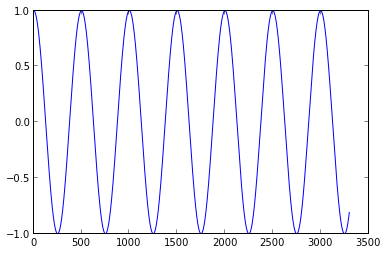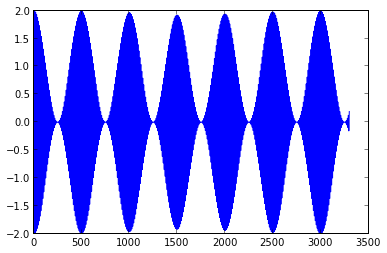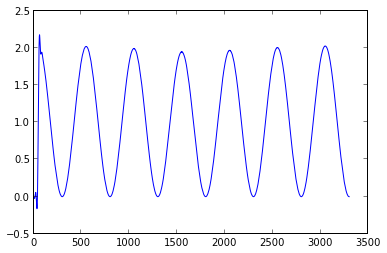In :
def modAMLarge(x, fc, phase, Ts):
t = linspace(0, len(x)*Ts, len(x))
c = cos(2*pi*fc*t+phase)

w = x*c+c
return w

def demodAMLarge(v, fc, Ts):
fbe = [0, 0.025, 0.05, 0.5]
damps = [1,0]
fl = 100
b = scipy.signal.remez(fl, fbe, damps)

envv = (pi/2)*scipy.signal.lfilter(b,1,abs(v))
return envv

time = 0.3333
Ts = 1.0/20000.0

t = linspace(0, time, time/Ts)

fm = 20.0
fc = 1000.0

x = 10/time*t+cos(2*pi*fm*t)

v = modAMLarge(x, fc, 0, Ts)
envv = demodAMLarge(v, fc, Ts)

plot(x)
figure()
plot(v)
figure()
plot(envv)

Out:
[<matplotlib.lines.Line2D at 0x113fd42d0>]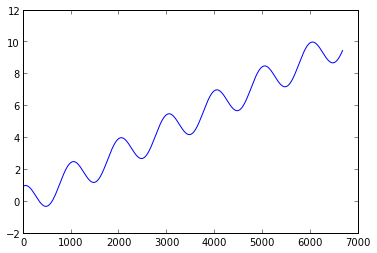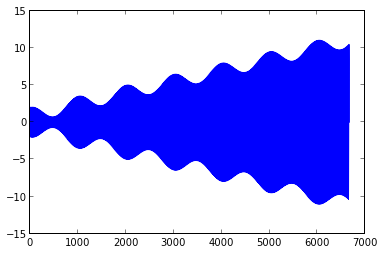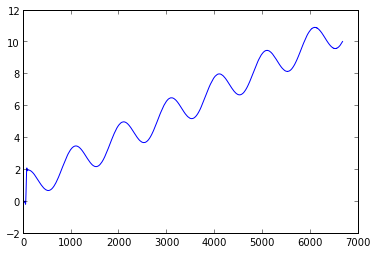5.1. Plot the spectrum of the message, the carrier, and the recieved signal. What is the spectrum of the envelope?

In :
time = 0.3333
Ts = 1.0/20000.0

t = linspace(0, time, time/Ts)

fm = 20.0
fc = 1000.0

x = 10/time*t+cos(2*pi*fm*t)

v = modAMLarge(x, fc, 0, Ts)
envv = demodAMLarge(v, fc, Ts)

plotspec(x, Ts)
plotspec(v, Ts)
plotspec(envv, Ts)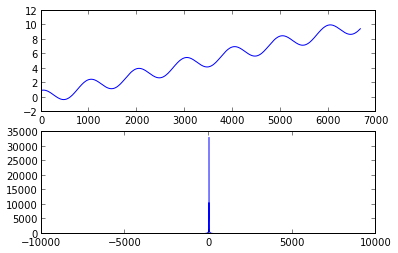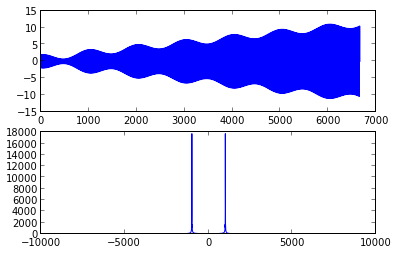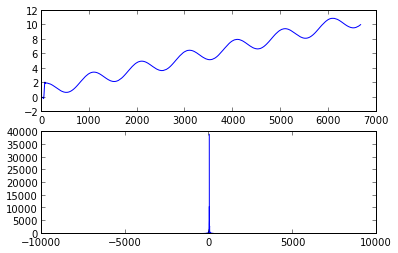5.2. One of the advantages of using large carrier AM is that you don't need the phase or frequency (exactly) of the transmitted signal. Verify

1. Change the phase of the transmitted signal, let c = cos(2pifc*t+phase) with phase = 0.1,0.5, pi/3, pi/2, pi
2. Change the frequency of the transmitted signal, and verify that the frequncy can vary by a bit
In :
def testRecovery(phase):
fc = 1000.0
Ts = 1.0/20000.0

v = modAMLarge(x, fc, 0, Ts)
envv = demodAMLarge(v, fc, Ts)
vp = modAMLarge(x, fc, phase, Ts)
envvp =  demodAMLarge(vp, fc, Ts)

return envvp-envv

d = testRecovery(0)
plot(d)

Out:
[<matplotlib.lines.Line2D at 0x113e71450>]